# ECE5725 Project：Biometric Guesser

Designed by
Brian Hsu (hh543) & Yitong Sun(ys555)

## Introduction

In this project we attempted to utilize these components …(list out all the components and images here).. Each of which will be discussed in further detail in the following sections. To tackle the problem mentioned in the objective section, three different biomedical sensors are tested using different methods and integrated into the system we designed. The three sensors are high accuracy temperature sensor, GSR sensor and pulse sensor, and their data are sent to an Arduino Uno. Then, the Uno transmits the data to Raspberry Pi 4. We intended to extract data from these biomedical sensors in real-time and then to do a decent judgment whether the person is lying or not. In order to show a straightforward result in the final demo, a simple game of guessing ball in cups was implemented and a dashboard of showing the nerves level was implemented both using the pygame library.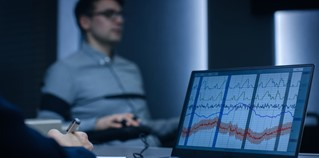Polygraph Test
Credit: Gorodenkoff/Shutterstock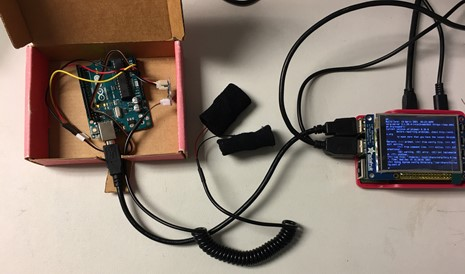System Overview

## Project Objective:

A polygraph, commonly known as a lie detector test, has been used as a way to tell if a person tells a lie or not. This project is to build an embedded system using raspberry pi and biomedical sensors to simulate such a device which can sense and detect whether the user is telling the truth or not. A simple game is also to be designed to demonstrate the result and judgment made by the system.

## Design and Testing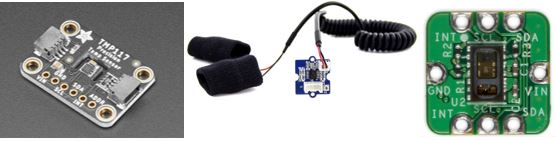Figure 3. Temperature Sensor, GSR Sensor and Pulse Sensor

Temperature Sensor

The temperature sensor worked perfectly fine with a high accuracy of 0.0001, and it communicated using I2C. We observed an issue with the temperature sensor, which is that the sensor has its own hardware temperature and usually starts with room temperature. It takes a while(usually 2-3 mins) to get heat equilibrium with the person’s finger. So, if the system wants to monitor the person’s body temperature and extract some pattern, it is not ready to use in a short time, which may affect the overall user experience of the system.

Establish Bluetooth Connection

The bluetooth component of the project involved communication between the Pi Zero and the Pi 4. The original intention is to hook up all the sensors onto the Pi Zero and communicate the data to the Pi 4 to achieve the concept of a lightweight wearable. This didn’t end up working out due to sensor integration reasons that will be discussed in the next section. Here, we will talk about successfully establishing the connection between the Zero and 4 via Bluetooth.
The Bluetooth module is needed to first be activated with the ‘rfkill unblock Bluetooth’ command, similar to what we did in Lab1 for WIFI. The status could be then confirmed with ‘systemctl status bluetooth’ as shown in the figure below. The command ‘hciconfig’ gives us the bd_addr, which is the bluetooth module identification address that will be needed in the python scripts to directly establish a RFCOMM connection.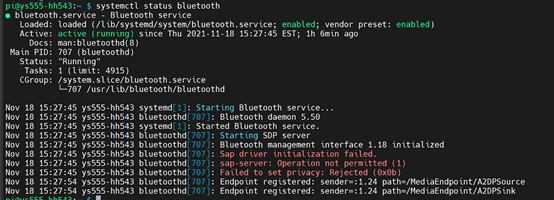Figure 4. RFCOMM Connection

On the Pi 4 as the server, we open up the Bluetooth socket and listen for communication to a specified port. For the Pi Zero as the client, the script initiates the connection with the given bd_addr and port number. Once the connection is established, data can be sent between the two devices. We modified the sample code below to keep the connection open with a while loop with the client sending commands to tell the server script what to do next.

GSR Hat to connect GSR sensor to Pi Zero

We ordered a hat for the GSR sensor because it uses an analog signal, which is not acceptable by RaspberryPi. The hat is also made by the same company as the GSR sensor, and theoretically, RPi can get correct data through the hat from the GSR sensor. We ran into lots of problems when we tried to install the software for the hat according to the provided tutorial. We tried the simple one-click installation at first but it didn’t work. Then, I tried a detailed step by step installation, but we were stuck at one of the dependency installations - Install MRAA & UPM. Prof and TA helped us debug and provided different ideas but still cannot fix it. As the GSR data is the most important data in this project that reflects human’s biomedical status, we decided to utilize Arduino Uno to collect all data from three sensors and to send the data to Pi4 using the serial port.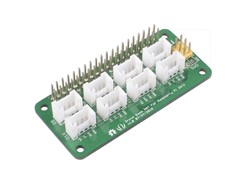Figure 5. Grove Hat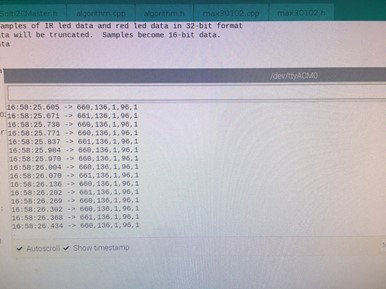Figure 6. Sensor Data on Arduino Serial Monitor

The GSR sensor worked well with Arduino and the communication between Arduino and Pi4 was also successfully built, which set all sensor data ready for use by the Pi4. The GSR sensor basically measures the conductivity of the skin. If a person gets nervous, he usually will sweat a little, which will result in the increase of skin conductivity. We first looked at the serial plotter in the Arduino IDE and tried to observe any patterns or features. The GSR data fluctuates a lot and we can only see the overall level change, so we decided to average the GSR data first in the Arduino code and care more about the overall level.

Arduino Uno to read sensor data and communicate with Pi 4

Figure 7 is the hardware diagram of the how the sensors are connected to the Arduino Uno. The maxrefdes117 pulse-oximeter is powered by the 3V port with the I2C pins connected to the arduino I2C ports and the interrupt connected to pin 10. The GSR sensor is powered by the 5V port and sends its data through the A0 analog port.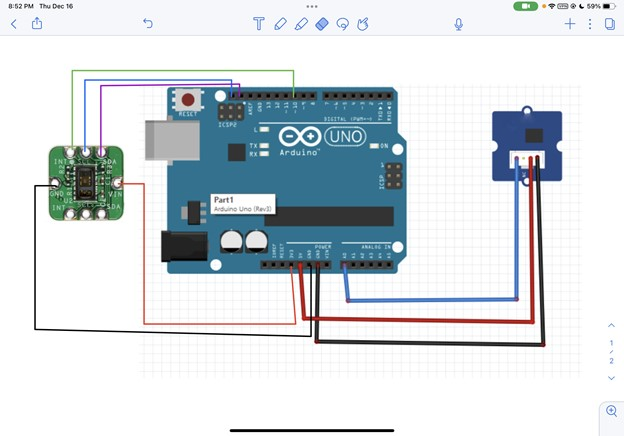Figure 7. Arduino Connection Layout

Figure 7 is the hardware diagram of the how the sensors are connected to the Arduino Uno. The maxrefdes117 pulse-oximeter is powered by the 3V port with the I2C pins connected to the arduino I2C ports and the interrupt connected to pin 10. The GSR sensor is powered by the 5V port and sends its data through the A0 analog port.

The next key part is to get communication working between the Arduino and the Pi4, especially for Pi4 reading data from Arduino using serial communication. We set up hardware permission for serial communication and installed the python serial library for Pi. The Arduino side platform would print the data through serial and the Pi 4 would read the serial line using the python serial library.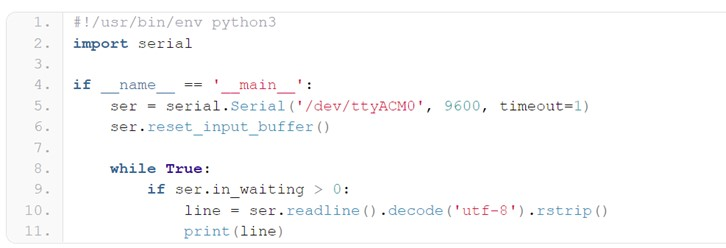Figure 8. Serial Communication Python Example

A test script for GSR level was written to observe the difference of GSR level when a person tells the truth and lies. For the first 20 secs, the person will be asked to speak out letters and numbers on the keyboard that are pointed and he must answer correctly. The GSR data average will be recorded and calculated. To be more specific, we use a sliding window average, which stores the average data from Arduino to a list and we calculate the last 20 elements in the list. Then, we will use these averages to calculate the final average for each 20 secs in both the calibration and testing stage. For another 20 secs, he will be asked the same questions but he must answer incorrectly. Also, the GSR data average will be recorded and calculated. A significant difference between two averages was observed, which will be mentioned in the results section.

We noticed that the absolute value of the sensor data varied between trials, thus we couldn’t simply compare the current value with a fixed baseline. Noticing that lie detection is more about the change of state, we decided to implement a calibration stage allowing us to measure the baseline of the user at the specific moment then compare the questioning measurements with said baseline.

The last sensor is the heart rate sensor. It turned out the sensor we received was just the sensor itself and not integrated onto a board for direct connection using jumper cables. We were able to borrow the maxrefdes117 integrated component from Sheryas to continue our work. We successfully extract heart rate data using Arduino code and send it to the Pi. The heart rate data had two outputs with the first one being the heart rate and the second one being the valid bit (Fig. 6). When testing, the heart rate sensor can reflect the basic level of heart rate according to whether a person is staying still calmly or jumping up and down, but it cannot differentiate when a person lies because 1) quick lies don’t cause that drastic of a change in heart rate 2) the computed heart rate fluctuates too much to confidently detect any slight changes in the heart rate

Game design and integration

Our goal is to use the percentage change from the baseline of these three sensors to calculate a stress score that determines the emotional shift of the user. We start by evaluating the three sensors separately to see their individual responses to the change in the user (i.e. lying in this case) to determine the weight each sensor should have on the overall stress score. With the characteristics of the sensors defined, we can represent and combine the data into an equation as shown. We could then integrate this score into our cup game and use it to determine the end results.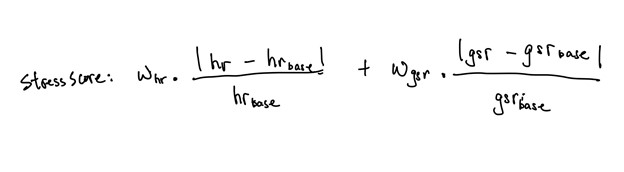Equation 1: Stress score calculation equation

As previously discussed, we noticed that the temperature sensor does not effectively capture helpful change so we decided not to include its data in the equation. We weighed GSR data more heavily since it is the most responsive to the lying condition. We weighed the heart rate significantly lower due to our observation of its inconsistent result despite no change in user status.

It is very tricky to do a proper demo game design to show our testing results. The general idea of the game is to divide the game into two parts. The first part is to set a baseline by asking some normal questions such as ‘Is the sky blue?’ The baseline data shows the person’s biomedical status when he is telling the truth. For the testing part, the baseline data can be used to judge whether he is lying or not.

We designed a cup game. The game process is that the player will answer a series of common sense questions correctly in the calibration stage. Then, he will choose one cup from four cups to hide the ball in. After that, he will be asked whether the ball is in a particular cup or not five times for a single cup. The player must answer no to all questions, which means that he will lie when he is asked if the ball is in the cup he chose previously.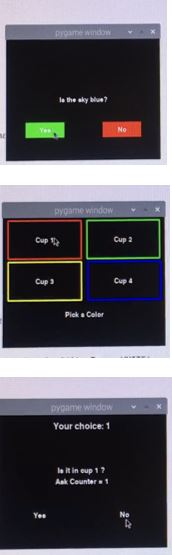Figure 9. Game Process

## Result

Lorem ipsum dolor sit amet, consectetur adipiscing elit. Vestibulum lorem nulla, consectetur at leo vel, pretium bibendum nisl. Cras blandit quam a enim ultrices, eu convallis enim posuere. Donec eleifend enim sed purus consectetur, vitae cursus lectus varius. Vivamus consectetur felis nec est venenatis posuere. Phasellus vitae aliquet erat. In laoreet lacinia mollis. Quisque iaculis nisl fermentum pharetra lobortis. Donec rhoncus dui sem, ac molestie leo tristique vel. Phasellus in nibh feugiat, fringilla lectus in, elementum magna. Etiam quis dui condimentum, tempus ex in, dapibus est. Cras ut congue augue. Donec ac enim ex. Ut id tristique risus, vel porttitor quam. Sed ultricies enim eu nibh porttitor, vel sodales enim feugiat. Fusce volutpat venenatis magna ac ultrices. Curabitur eget urna ut nulla mattis convallis non eu diam.

A baseline data will be calculated during the calibration stage. Four averages will also be recorded and calculated for four different cups when the player is asked questions. An overall score can be generated by the data from GSR sensor and heart rate sensor. A judgment will be made by comparing the baseline average and four different cup question averages. According to the test we made, the lower the score is, the more possible the ball is in that cup.

The overall results left much to be desired. We envisioned a light-weight wearable capable of differentiating emotional status and detecting any lie. We hoped to demonstrate the effectiveness of our sensor data by incorporating the data in the form of a “find the ball” cup game implemented through pygame. We were able to successfully create the pygame interface that incorporated an overall game flow from calibration, in-game questioning, result display and show a person’s emotional status through visual representation in the form of a stress meter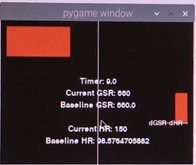Figure 10. Stress Score Monitoring Screen

The issues we came across could be roughly categorized into two sections:
1. Human Variability
2.Sensor consistency

Human Variability

The core concept of our project predicated on the sensor being able to pick up the autonomic arousal of the user. This required us to first experiment what conditions could trigger noticeable change in the sensor reading.

We found out that human biometric status does not vary significantly with isolated yes/no questions as there is neither enough weight behind the question to trigger a significant emotional response nor a long enough exposure time for the sensor to pick up the changes. When considering how to ask the questions, we realize that an open-ended format (i.e. describing a true and false story) potentially introduced many human factors that cannot be quantified, thus we chose a more straightforward approach in asking the user to identify the keys on a keyboard (i.e. first truthfully, then falsely). With the “describe a true/false story” calibration method, we saw a significant difference in GSR readings with the false story showing a lower reading. The more controlled calibration method (i.e. keyboard key identification) showed results differentiating between ±10-20 in GSR reading (Fig. 10), which was also a significant difference considering that these results are the average of the sliding window measurements.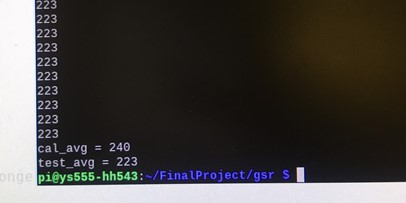Figure 11. GSR Calibration Test (keyboard keys identification)

We noticed that our questioning options were limited in game (e.g. asking which cup) which made it more difficult to trigger a significant response, while accounting for other human factors such as anticipation and engagement. Using repetition of the same question, we were able to lengthen the duration of any particular state that the user was in and allow the sensor to differentiate truth and lie. This was determined heavily by the GSR as we noticed that heart rate data doesn’t change noticeably. Figure 11 is the result screen of one of the test runs where we chose cup 1 to hide the ball in. We can see that the GSR reading for cup 1 does indeed appear to be the lowest. However, we notice that the difference is not as significant with only a ±5 in reading.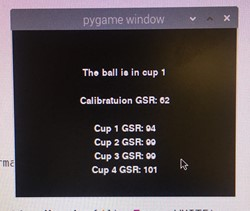Figure 12. Cups game Result Screen

Sensor Consistency

Going back to figure 11, the calibration GSR value is much lower than all the other values. We’ve noticed that throughout our various experiments the sensors are extremely sensitive to any changes in the environment. For example, if we were to put our hands on the ESD pad while running measurements this would drastically shift the outputting values. Another example is if we use the fingers on the same hand to activate both the GSR sensor and pulse-oximeter, the measurements will become skewed (i.e. this is verified by lifting the finger on the pulse-oximeter off). As for the pulse-oximeter, any slight pressure change or shift of the finger could cause a change in the measurements. We can see from figure 12that the heart rate fluctuates between two 15 second intervals. This is why we impose a strict calibration check (i.e. requiring two consecutive measurements to be within ±10) before moving onto the biometric dashboard. We observed that there are a lot of factors that could cause interference with the reading. Averaging the results from the sensor helped stabilize the sensor output to a degree where we could observe an overall trend in rise (i.e. heart rate increase from jumping up and down) or fall (i.e. GSR drop from user lying) that is due to the user and not ambient interference.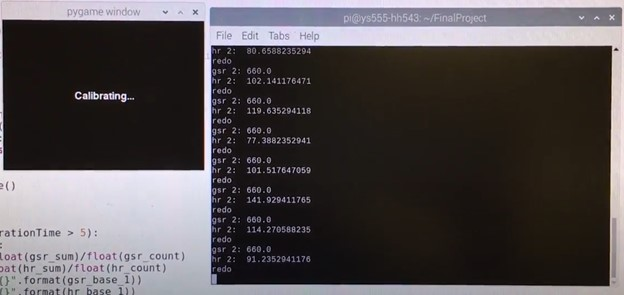Figure 13. Stress Score Interface Calibration Stage

## Conclusions

Our project achieves the capability of sensing the heart rate and GSR changes stimulated from physical exertion and long duration lying. Although the resolution of such detection is under significant external interferences, we were able to mitigate some of the interference with various averaging methods and weight calculation.

Overall, we believe that there were still a lot of options to explore in order to create a more accurate and stable lie detector as the results were still fairly inconsistent. The obstacles we’ve encountered illustrated the difficulty of embedded systems design and integration due to the continuous update of the Pi systems. Our experience with this project showed that it is not impossible to design a much more consistent lie detector but much more factors would need to be taken into consideration.

I believe we relied too heavily on the arduino library for the pulse-oximeter which had an algorithm for calculating the heart rate. We were trying to come up with a questioning scheme and tinkering with the weight contribution for each sensor but it’s generally not a good approach to try and make a broken system work. Although it was a bit too late, we realized that the reason the sensor results (i.e. specifically the heart rate data) was so jumpy might be due to the lack of memory of the Arduino Uno board. Therefore, the limited sampling points used to compute the Fourier transform to obtain the heart rate were not sufficient in providing a consistent result despite the user remaining still.

## Future Work

As mentioned in the conclusion, we would like to implement our own signal processing algorithm from scratch. Instead of doing the processing on the Uno board, we would transmit the raw data from the pulse-oximeter to the Pi 4. Making use of the amazing computing power and memory of the Pi 4 along with the SciPy library, we can calculate a more consistent heart rate via the discrete Fourier transform of a much larger sampling pool. On top of that, we could implement a filtering system that takes care of the interferences caused by users shifting their finger.

On the questioning scheme side of things, we could definitely work on a more well-rounded method that extracts as much human factors as possible. One thought is we should’ve randomized the cup at which the computer chooses to ask to minimize the effects of human anticipation. A more extensive exploration would be to implement voice recognition. This allows us to go beyond yes/no questions and even possibilities of analyzing the confidence of one’s voice.

## Diagram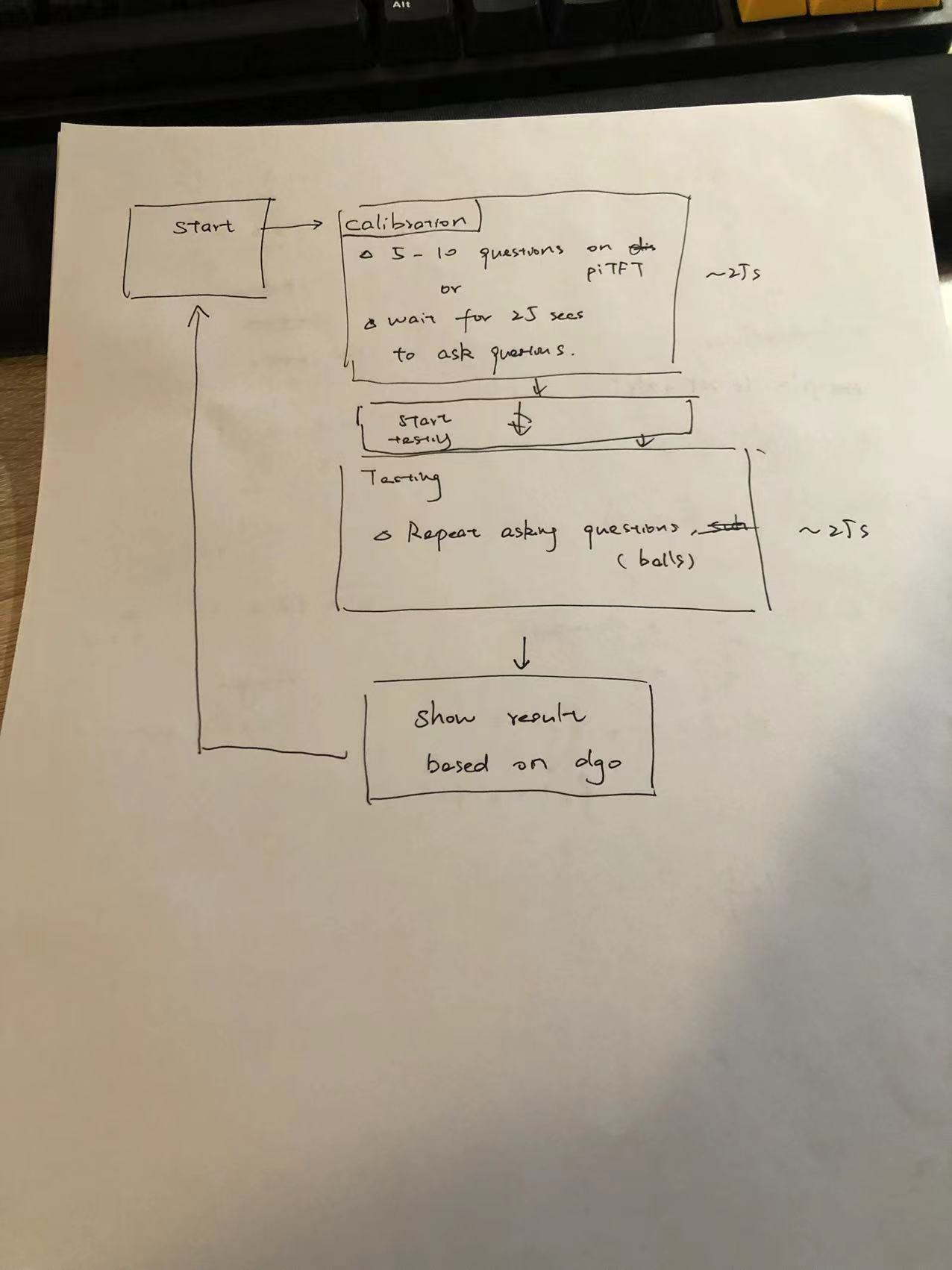Figure 14. Game Flowchart

## Work Distribution### Brian Hsu

hh543@cornell.edu

Pygame design and integration with pulse-oximeter sensor, Data processing of sensor data, Bluetooth communication, Game flow design### Yitong Sun

ys555@cornell.edu

Polygraph paper research, Data processing of sensor data, Arduino communication,, Game flow design.

## References

Temperature Sensor Guide
GSR with Arduino
UART Communication
Pulse Sensor
Bluetooth Wrapper
Typical Polygraph Procedure

## Code Appendix

gameDisplay.py

```import pygame, os
from pygame.locals import *
import time
import serial
# import RPi.GPIO as GPIO

#setup environment to run on piTFT
#os.putenv('SDL_VIDEODRIVER', 'fbcon')
#os.putenv('SDL_FBDEV', '/dev/fb1')
#os.putenv('SDL_MOUSEDRV', 'TSLIB')
#os.putenv('SDL_MOUSEDEV', '/dev/input/touchscreen')

# GPIO.setmode(GPIO.BCM)
# GPIO.setup(27, GPIO.IN, pull_up_down=GPIO.PUD_UP)
# GPIO.setup(23, GPIO.IN, pull_up_down=GPIO.PUD_UP)
# def GPIO27_callback(channel):
#     global inGame
#     inGame = False

# startGame = False
# def GPIO23_callback(channel):
#     # start the game
#     global startGame
#     startGame = True

ser = serial.Serial('/dev/ttyACM0',9600, timeout = 10)
ser.reset_input_buffer()
# log file
#f = open('test.txt', 'r')

pygame.init()
size = width, height = 320, 240
BLACK = 0, 0 ,0
WHITE = 255,255,255
RED = 255, 0, 0
GREEN = 0, 255, 0
BLUE = 0, 0, 255
YELLOW = 255,255,0

screen = pygame.display.set_mode(size)
font = pygame.font.Font(None, 20)
font_24 = pygame.font.Font(None, 24)
# FLAGS
inGame = True
landingPage = True
calibrationStage = False
pickStage = False
questionStage = False
resultStage = False
settingRelaxedBaseline = False
settingAnxiousBaseline = False

### calibration data structures and functions
qArr = []
aArr = []
f = open('Q&A.txt', 'r')
for i in range(len(x)):
x[i] = x[i][:-2]
qArr = x[::2]
aArr = x[1::2]

# VARIABLES
totalQs = len(qArr)
yesClicked = True
correct = 0
incorrect = 0

global aArr, answeredQs, totalQs, yesClicked, correct, incorrect, calibrationStage, pickStage
yesCorrect = yesClicked and ans == 'Yes'
noCorrect = not yesClicked and ans == 'No'
if(yesCorrect or noCorrect):
correct = correct + 1
else:
incorrect = incorrect + 1
calibrationStage = False
pickStage = True
recordCalibration()
draw4CupsScreen()

### testing stage data structures and functions
choice = 0

# basic design

# Alt design

txt = font_24.render('Your choice: {}'.format(choice), True, WHITE)
rect = txt.get_rect(center=(160, 20))
screen.blit(txt,rect)
txt = font.render('Is it in cup {} ? '.format(askCup), True, WHITE)
rect = txt.get_rect(center=(160,100))
screen.blit(txt, rect)
rect = txt.get_rect(center=(160,120))
screen.blit(txt, rect)

### drawing functions
def drawResultScreen():
global cupMeanArr, calibration_gsr
#     txt = font.render('Results', True, WHITE)
#     rect = txt.get_rect(center=(80,180))
#     screen.blit(txt, rect)
resultCup = 0
currMin = cupMeanArr
for i in range(len(cupMeanArr)-1):
if(cupMeanArr[i] < currMin):
currMin = cupMeanArr[i]
resultCup = i

txt = font.render('The ball is in cup {}'.format(resultCup + 1), True, WHITE)
rect = txt.get_rect(center=(160,60))
screen.blit(txt, rect)

txt = font.render('Calibratuion GSR: {}'.format(cupMeanArr), True, WHITE)
rect = txt.get_rect(center=(160,100))
screen.blit(txt, rect)
txt = font.render('Cup 1 GSR: {}'.format(cupMeanArr), True, WHITE)
rect = txt.get_rect(center=(160,140))
screen.blit(txt, rect)
txt = font.render('Cup 2 GSR: {}'.format(cupMeanArr), True, WHITE)
rect = txt.get_rect(center=(160,160))
screen.blit(txt, rect)
txt = font.render('Cup 3 GSR: {}'.format(cupMeanArr), True, WHITE)
rect = txt.get_rect(center=(160,180))
screen.blit(txt, rect)
txt = font.render('Cup 4 GSR: {}'.format(cupMeanArr), True, WHITE)
rect = txt.get_rect(center=(160,200))
screen.blit(txt, rect)
def draw4CupsScreen():
global cup1, cup2, cup3, cup4
cup1 = pygame.draw.rect(screen, RED, (10,5 ,145,72.5), 3)
cup2 = pygame.draw.rect(screen, GREEN, (165,5,145,72.5), 3)
cup3 = pygame.draw.rect(screen, YELLOW, (10,82.5,145,72.5), 3)
cup4 = pygame.draw.rect(screen, BLUE, (166,82.5,145,72.5), 3)

txt = font.render('Cup 1', True, WHITE)
rect = txt.get_rect(center=(72.5+10,5+72.5/2))
screen.blit(txt, rect)
txt = font.render('Cup 2', True, WHITE)
rect = txt.get_rect(center=(145+72.5+20,5+72.5/2))
screen.blit(txt, rect)
txt = font.render('Cup 3', True, WHITE)
rect = txt.get_rect(center=(72.5+10,10+72.5+72.5/2))
screen.blit(txt, rect)
txt = font.render('Cup 4', True, WHITE)
rect = txt.get_rect(center=(145+72.5+20,10+72.5+72.5/2))
screen.blit(txt, rect)
txt = font.render('Pick a Color', True, WHITE)
rect = txt.get_rect(center=(160,180))
screen.blit(txt, rect)

def drawYesNo2():
global yesButton2, noButton2
txt = font.render('Yes', True, WHITE)
yesButton2 = txt.get_rect(center=(80,180))
screen.blit(txt, yesButton2)
txt = font.render('No', True, WHITE)
noButton2 = txt.get_rect(center=(240,180))
screen.blit(txt, noButton2)
def drawYesNo():
pygame.draw.rect(screen, GREEN, (40, 165, 80, 30))
pygame.draw.rect(screen, RED, (200, 165, 80,30))
global yesButton, noButton
txt = font.render('Yes', True, WHITE)
yesButton = txt.get_rect(center=(80,180))
screen.blit(txt, yesButton)
txt = font.render('No', True, WHITE)
noButton = txt.get_rect(center=(240,180))
screen.blit(txt, noButton)

def drawQuestion():
if(calibrationStage):
questionFont = font.render(question, True, WHITE)
rect = questionFont.get_rect(center=(160,120))
screen.blit(questionFont, rect)

def displayFrontPage():
global startButton
startButton = pygame.draw.rect(screen, GREEN, (80,180,160,30))
gameTitle = font.render('Biometric Guesser', True, WHITE)
rect = gameTitle.get_rect(center=(160,80))
screen.blit(gameTitle, rect)

txt = font.render('Start', True, WHITE)
rect = txt.get_rect(center=(160,195))
screen.blit(txt, rect)

#     measuredData = font.render(data, True, WHITE)
#     rect = measuredData.get_rect(center=(160, 40))
#     screen.blit(measuredData, rect)
gsr_val = 0
hr_val = 0
bo_val = 0
def displaySensorData():
global gsr_val, hr_val, bo_val
txt = font.render("GSR: {}".format(gsr_val), True, WHITE)
rect = txt.get_rect(center=(80,80))
screen.blit(txt, rect)
txt = font.render("Heart Rate: {}".format(hr_val), True, WHITE)
rect = txt.get_rect(center=(80,140))
screen.blit(txt, rect)
txt = font.render("Blood O2: {}".format(bo_val), True, WHITE)
rect = txt.get_rect(center=(80,220))
screen.blit(txt, rect)

gsr_count = 0
gsr_sum = 0
gsr_mean = 0
hr_arr = []
bo_arr = []
def recordCalibration():
global gsr_count, gsr_sum, gsr_mean
gsr_mean = gsr_sum/gsr_count
cupMeanArr = gsr_mean
print(gsr_mean)
def clearData():
global gsr_count, gsr_sum, gsr_buffer
gsr_count = 0
gsr_sum = 0
gsr_buffer = []
cupMeanArr = [0,0,0,0,0]
def storeCupMean():
gsr_buffer = []
hr_buffer = []

warmTime = time.time()
warmFlag = True
while(warmFlag):
if ser.in_waiting > 0:
data = value.split(',')
if(len(data) == 5):
warmFlag = False

while inGame:
if ser.in_waiting > 0:
data = line.split(',')
gsr_val = int(data)
hr_val = int(data)
hr_t = int(data)
bo_val = int(data)
bo_t = int(data)
print(gsr_val)
print(hr_val)
print(hr_t)
print(bo_val)
print(bo_t)

while(len(gsr_buffer) < 15):
gsr_buffer.append(gsr_val)

gsr_buffer.append(gsr_val)
gsr_buffer =gsr_buffer[-15:]
avg = sum(gsr_buffer)/15

gsr_sum += avg
gsr_count += 1
print(avg)

# update screen

for event in pygame.event.get():
if(event.type == pygame.MOUSEBUTTONDOWN):
pos = pygame.mouse.get_pos()
print('click')
elif(event.type == pygame.MOUSEBUTTONUP):
pos = pygame.mouse.get_pos()
x, y = pos
if(landingPage):
if(startButton.collidepoint(x,y)):
landingPage = False
#set configure page required variables
#settingRelaxedBaseline = True
calibrationStage = True
drawYesNo()
#clicked yes
if(calibrationStage):
if(yesButton.collidepoint(x,y)):
yesClicked = True
print('pressed yes')
#clicked no
if(noButton.collidepoint(x,y)):
yesClicked = False
print('pressed no')

if(pickStage):
if(cup1.collidepoint(x,y)):
choice = 1
clearData()
pickStage = False
questionStage = True
drawYesNo2()
if(cup2.collidepoint(x,y)):
choice = 2
clearData()
pickStage = False
questionStage = True
drawYesNo2()
if(cup3.collidepoint(x,y)):
choice = 3
clearData()
pickStage = False
questionStage = True
drawYesNo2()
if(cup4.collidepoint(x,y)):
choice = 4
clearData()
pickStage = False
questionStage = True
drawYesNo2()

# testing stage
if(questionStage):
if(yesButton2.collidepoint(x,y)):
#clearData()
clearData() #clear data at the beginning of asking a new cup
storeCupMean()

if(askCup == 5): # end the stage
questionStage = False
resultStage = True

if(noButton2.collidepoint(x,y)):
#clearData()
clearData()
storeCupMean()

if(askCup == 5): # end the stage
questionStage = False
resultStage = True
elif event.type == pygame.KEYDOWN:
if event.key == pygame.K_LEFT:
pygame.quit()
quit()
#clicked yes
# draw updated screen
screen.fill(BLACK)
if(landingPage):
#displaySensorData()
displayFrontPage()
#drawYesNo2()
#draw4CupsScreen()
if(calibrationStage):
drawQuestion()
drawYesNo()

if(pickStage):
draw4CupsScreen()
if(questionStage):
drawYesNo2()
if(resultStage):
drawResultScreen()

pygame.display.flip()

#GPIO.cleanup()
```

dashboardDisplay.py

```# v5
# want to add individaul horizontal offset meters as well as interrupt to reinitiate calibrate sequence
# and test if larger averaging window gives better results
import pygame, math
from pygame.locals import *
import time
import serial
#import RPi.GPIO as GPIO

#setup environment to run on piTFT
# os.putenv('SDL_VIDEODRIVER', 'fbcon')
# os.putenv('SDL_FBDEV', '/dev/fb1')
# os.putenv('SDL_MOUSEDRV', 'TSLIB')
# os.putenv('SDL_MOUSEDEV', '/dev/input/touchscreen')

# GPIO.setmode(GPIO.BCM)
# GPIO.setup(27, GPIO.IN, pull_up_down=GPIO.PUD_UP)
# # GPIO.setup(23, GPIO.IN, pull_up_down=GPIO.PUD_UP)
# def GPIO27_callback(channel):
#     GPIO.cleanup()
#     quit()

# serial ports
ser = serial.Serial('/dev/ttyACM0',9600, timeout = 10)
ser.reset_input_buffer()

BLACK = 0, 0 ,0
WHITE = 255,255,255
pygame.init()
pygame.font.init()
screen = pygame.display.set_mode((320,240))
font_16 = pygame.font.Font(None, 16)
font_20 = pygame.font.Font(None, 20)
font_24 = pygame.font.Font(None, 24)

# the best way to animate the bar is first have parameters
# parameters:
# min: 0.7 of baseline ; Max 1.7 from baseline
class Bar():
def __init__(self, gsr_baseline, hr_baseline, bias, ):
self.gsr_baseline = gsr_baseline
self.hr_baseline = hr_baseline
# self.right_length = 50 #pixels
# self.left_length = 50 #pixels
self.unitLength = 10 #unit (dial) #pixel
self.totalLength = 200
self.bias = bias #could adjust this. Not used
self.dial = self.gsr_baseline/self.gsr_baseline - 1
self.color = WHITE

self.hr_dial = 0
self.hr_color = WHITE
self.gsr_dial = 0
self.gsr_color = WHITE

self.colorArr = [(254, 240, 1), (255, 206, 3), (253, 154, 1), (253, 97, 4), (255, 44, 5), (240, 5, 5)]
self.currGSR = 0
self.currHR = 0

# I should adjust baseline every 5 minutes or something
# not used yet (integrate as a score)
def updateBaseline(self, gsr_baseline, hr_baseline):
self.gsr_baseline = gsr_baseline
self.hr_baseline = hr_baseline
self.dial = self.gsr_baseline/self.gsr_baseline - 1

def updateDial(self, currGSR, currHR):
self.currGSR = currGSR
self.currHR = currHR
self.dial = (1/1)*abs(currHR-self.hr_baseline)/hr_baseline
#self.dial = 2*abs(currGSR - self.gsr_baseline)/self.gsr_baseline + (1/1)*abs(currHR-self.hr_baseline)/hr_baseline#(input/self.baseline) -1

print("baseline = {}".format(gsr_baseline))
print("dial = {}".format(self.dial))
colorIdx = int(math.floor(abs(self.dial*50/2)))
if(colorIdx > len(self.colorArr) - 1):
colorIdx = len(self.colorArr) - 1
self.color = self.colorArr[colorIdx]

#individual gsr, hr
self.gsr_dial = (currGSR - self.gsr_baseline)/ self.gsr_baseline
colorIdx = int(math.floor(abs(self.gsr_dial*50/2)))
if(colorIdx > len(self.colorArr) - 1):
colorIdx = len(self.colorArr) - 1
self.gsr_color = self.colorArr[colorIdx]
self.hr_dial = (currHR - self.hr_baseline)/hr_baseline
colorIdx = int(math.floor(abs(self.hr_dial*50/2)))
if(colorIdx > len(self.colorArr) - 1):
colorIdx = len(self.colorArr) - 1
self.gsr_color = self.colorArr[colorIdx]

def displayBar(self):
sizingCoefficient = 10
wOffset = abs(self.dial*self.totalLength)

if(wOffset > 200):
wOffset = 200
pygame.draw.rect(screen, self.color, (10, 10,abs(wOffset),50))
# display individual bars
gsr_h_offset = (self.currGSR - self.gsr_baseline)*self.unitLength
hr_h_offset = (self.currHR - self.hr_baseline)*self.unitLength
#gsr
start = 170 #120 - 220
if(gsr_h_offset < -50):
gsr_h_offset = -50
elif(gsr_h_offset > 50):
gsr_h_offset = 50
if(gsr_h_offset >0):
start = start + (-gsr_h_offset)
pygame.draw.rect(screen, self.gsr_color, (260, start,20, abs(gsr_h_offset)))
txt = font_16.render('dGSR', True, WHITE)
rect = txt.get_rect(center=(260,170))
screen.blit(txt, rect)
#hr
start = 170 #120 - 220
hr_h_offset
if(hr_h_offset < -50):
hr_h_offset = -50
elif(hr_h_offset > 50):
hr_h_offset = 50
if(hr_h_offset > 0):
start = start + (-hr_h_offset)
pygame.draw.rect(screen, self.gsr_color, (290, start,20, abs(hr_h_offset)))
txt = font_16.render('dHR', True, WHITE)
rect = txt.get_rect(center=(290,170))
screen.blit(txt, rect)

#pygame.draw.rect(screen, self.hr_color, (10, start,20,abs(hOffset)))

### version 2
#         wOffset = self.dial*self.length
#         center = 160
#         start = center
#         if(wOffset < 0):
#             start = start + wOffset
#         pygame.draw.rect(screen, self.color, (start, 10,abs(wOffset),50))

def displayData(self):
global startTime
diff = round(time.time() - startTime)
txt = font_20.render('Timer: {}'.format(diff), True, WHITE)
rect = txt.get_rect(center=(160,100))
screen.blit(txt, rect)
txt = font_20.render('Current GSR: {}'.format(self.currGSR), True, WHITE)
rect = txt.get_rect(center=(160,120))
screen.blit(txt, rect)
txt = font_20.render('Baseline GSR: {}'.format(self.gsr_baseline), True, WHITE)
rect = txt.get_rect(center=(160,140))
screen.blit(txt, rect)
txt = font_20.render('Current HR: {}'.format(self.currHR), True, WHITE)
rect = txt.get_rect(center=(160,180))
screen.blit(txt, rect)
txt = font_20.render('Baseline HR: {}'.format(self.hr_baseline), True, WHITE)
rect = txt.get_rect(center=(160,200))
screen.blit(txt, rect)

buffCounter = 0
def drawBuffering():
global buffCounter
var = buffCounter % 3
txt = font_24.render('Calibrating...', True, WHITE)
rect = txt.get_rect(center=(160,120))
screen.blit(txt, rect)

#     if(var == 1 or var==2):
#         txt = font_24.render('.', True, WHITE)
#         rect = txt.get_rect(center=(162,195))
#         screen.blit(txt, rect)
#     if(var == 2):
#         txt = font_24.render('Buffering', True, WHITE)
#         rect = txt.get_rect(center=(164,195))
#         screen.blit(txt, rect)
def drawContinue():
txt = font_24.render('Tap to Continue', True, WHITE)
rect = txt.get_rect(center=(160,195))
screen.blit(txt, rect)
def cleanSensorVar():
global gsr_buffer, gsr_sum, gsr_count, hr_sum, hr_count
gsr_sum = 0
gsr_count = 0
hr_sum = 0
hr_count = 0

# gsr data variables
gsr_buffer = []
gsr_count = 1
gsr_sum = 1
gsr_base_1 = 0
gsr_base_2 = 0
gsr_size = 15
# hr data variables
hr_buffer = []
hr_size = 15
hr_count = 0
hr_sum = 0
hr_base_1 = 0
hr_base_2 = 0

calib1done= False
calib2done = False
bufferStage = False
calibrationStage = True
# I want to give time for arduino to warm up but also display buffering stage
# bufferTime = time.time()
# while bufferStage:
#     if(time.time() - bufferTime > 10):
#         bufferStage = False
#         calibrationStage = True
#     screen.fill(BLACK)
#     drawBuffering()
#     pygame.display.flip()

#intentional delay stage for the arduino to start transmitting
warmTime = time.time()
warmFlag = True
while(warmFlag):
if ser.in_waiting > 0:
data = value.split(',')
if(len(data) == 5):
warmFlag = False

calibrationTime = time.time()
delayTime = time.time()
bufferTime = time.time()
while calibrationStage:
if(time.time() - calibrationTime > 5):
if(not calib1done):
gsr_base_1 = float(gsr_sum)/float(gsr_count)
hr_base_1 = float(hr_sum)/float(hr_count)
print("gsr 1: {}".format(gsr_base_1))
print("hr 1:  {}".format(hr_base_1))
calib1done = True
cleanSensorVar()
calibrationTime = time.time() #reset calibration timer
elif (not calib2done):
gsr_base_2 = float(gsr_sum)/float(gsr_count)
hr_base_2 = float(hr_sum)/float(hr_count)
print("gsr 2: {}".format(gsr_base_2))
print("hr 2:  {}".format(hr_base_2))
calib2done = True
if(abs(gsr_base_1 - gsr_base_2) > 10 or abs(hr_base_1 - hr_base_2) > 10): #calibration differece too large means not calibrated
gsr_base_1 = gsr_base_2
hr_base_1 = hr_base_2
calib2done = False
cleanSensorVar()
calibrationTime = time.time() #reset calibration timer
print('redo')
else:
gsr_baseline = gsr_base_2
hr_baseline = hr_base_2
bar = Bar(gsr_baseline, hr_baseline, 0.3) # bias is unused rn
calibrationStage = False
# Choose not to clean gsr variables means calibration data will influence (I should at least clean sum & count)
gsr_buffer = len(gsr_buffer)*[gsr_baseline]
gsr_sum = 0
gsr_count = 0
hr_buffer = len(hr_buffer)*[hr_baseline]
hr_sum = 0
hr_count = 0
if ser.in_waiting > 0:
data = line.split(',')
if( len(data) == 5): #els
#if(time.time() - delayTime > 1 and len(data) == 5): #else just read it off the serial and send it into the void
gsr_val = int(data)
hr_val = int(data)
hr_t = int(data)
bo_val = int(data)
bo_t = int(data)

#
if(len(gsr_buffer) < gsr_size or len(hr_buffer) < hr_size):
gsr_buffer.append(gsr_val)
gsr_buffer = gsr_buffer[-gsr_size:]
if(hr_t):
hr_buffer.append(hr_val)

else: # if this part was never entered due to not enough valid hr data, the count would be zero which would cause exception
gsr_buffer.append(gsr_val)
gsr_buffer =gsr_buffer[-gsr_size:]
avg = sum(gsr_buffer)/gsr_size
gsr_sum += avg
gsr_count += 1
# HR data
if(hr_t):
hr_buffer.append(hr_val)
hr_buffer= hr_buffer[-hr_size:]
hr_avg = sum(hr_buffer)/hr_size
hr_sum += hr_avg
hr_count += 1
#                 print(avg) # gsr at time instant

screen.fill(BLACK)
#     if(time.time() - bufferTime > 0.2):
#         buffCounter += 1
drawBuffering()
pygame.display.flip()
waitStage = True
while(waitStage):
for event in pygame.event.get():
if(event.type == pygame.MOUSEBUTTONDOWN):
pos = pygame.mouse.get_pos()
elif(event.type == pygame.MOUSEBUTTONUP):
pos = pygame.mouse.get_pos()
x, y = pos
print('click')
waitStage = False
break
screen.fill(BLACK)
drawContinue()
#     if(time.time() - blinkTime > 0.2):
#         drawContinue()
pygame.display.flip()
#### phase 2 ########
startTime = time.time()
#testing
dialTime = time.time() #this is the basically the time frame to update the bar display
arr = [11, 12, 13, 14, 15, 16, 17, 18, 19, 20, 21, 22, 23, 24, 25 ,26]
i = 0
newDialVal = arr[i]

while True:

# simulate refreshing baseline to monitor fairly recent status
if(time.time()-startTime > 120):
gsr_base_new = float(gsr_sum)/float(gsr_count)
hr_base_new = float(hr_sum)/float(hr_count)
bar.updateBaseline(gsr_base_new, hr_base_new) # I'm trying to feed in
cleanSensorVar()
startTime = time.time()

if ser.in_waiting > 0:
data = line.split(',')
if(len(data) == 5): #else just read it off the serial and send it into the void
gsr_val = int(data)
hr_val = int(data)
hr_t = int(data)
bo_val = int(data)
bo_t = int(data)

gsr_buffer.append(gsr_val)
gsr_buffer =gsr_buffer[-gsr_size:]
gsr_avg = sum(gsr_buffer)/gsr_size # for display

if(hr_t):
hr_buffer.append(hr_val)
hr_buffer= hr_buffer[-hr_size:]

hr_avg = sum(hr_buffer)/hr_size

bar.updateDial(gsr_avg, hr_avg)

# for next baseline computation
gsr_sum += gsr_avg
gsr_count += 1
hr_sum += hr_avg
hr_count += 1
#simulate dial change from sensor data input
#         if(time.time() - dialTime > 0.5):
#             i = i+ 1
#             idx = i%len(gsr_buffer)
#
# #             idx = i%len(arr)
# #
# #             newDialVal = arr[idx]
# #             dialTime = time.time()
# #             bar.updateDial(newDialVal)

for event in pygame.event.get():
if(event.type == pygame.MOUSEBUTTONDOWN):
pos = pygame.mouse.get_pos()
elif(event.type == pygame.MOUSEBUTTONUP):
pos = pygame.mouse.get_pos()
x, y = pos
elif event.type == pygame.KEYDOWN:
if event.key == pygame.K_LEFT:
pygame.quit()
quit()

screen.fill(BLACK)
# change the color as dial strays away from baseline

pygame.draw.line(screen, WHITE, (160,0), (160,240))
pygame.draw.line(screen, WHITE, (260,170),(280,170))
pygame.draw.line(screen, WHITE, (290,170),(310,170))
bar.displayBar()
# draw current values and baseline value
bar.displayData()
# pygame.draw.rect(screen, WHITE, (0, 0,160,80))
```

gsr_hr_log.py

```#!/user/bin/env python3

# 2021/11/30 Code to test gsr on arduino to pi4 vis uart serial

import serial
import sys
import time
#import matplotlib.pyplot as plt

if __name__ == '__main__':
ser = serial.Serial('/dev/ttyACM0',9600, timeout = 10)
ser.reset_input_buffer()

buffer = []
cal_sum = 0
cal_count = 0
test_sum = 0
test_count = 0
buffer_2 = []
hr_buffer = []
hr_buffer_2 = []
hr_cal_sum = 0
hr_cal_count = 0
hr_test_sum = 0
hr_test_count = 0

startTime = time.time()
if(len(sys.argv) == 2):
version = int(sys.argv)
f = open("logs/log_v{}".format(version), 'w')
# proceed with program
print('y to start Calibrate')
x = input()
f.write('calibration.................\n')
while(len(buffer) < 20):
if ser.in_waiting > 0:
data = value.split(',')
gsr_val = int(data)
hr_val = int(data)
hr_t = int(data)
bo_val = int(data)
bo_t = int(data)
buffer.append(gsr_val)

while len(hr_buffer) < 5:
if ser.in_waiting > 0:
data = value.split(',')
gsr_val = int(data)
hr_val = int(data)
hr_t = int(data)
bo_val = int(data)
bo_t = int(data)

if hr_t == 1:
hr_buffer.append(hr_val)

while time.time()-startTime < 15:  # calibration time might be a little longer
if ser.in_waiting > 0:
data = value.split(',')
gsr_val = int(data)
hr_val = int(data)
hr_t = int(data)
bo_val = int(data)
bo_t = int(data)
buffer.append(gsr_val)
buffer = buffer[-20:]
avg = sum(buffer)/20
f.write(str(avg) + '\n')
# HR
hr_buffer.append(hr_val)
hr_buffer = hr_buffer[-5:]
hr_avg = sum(hr_buffer)/5
# HR cal_avg
hr_cal_sum += hr_avg
hr_cal_count += 1

# get total cal_avg
cal_sum += avg
cal_count += 1
#print(avg)
print(hr_avg)
cal_avg = cal_sum / cal_count
hr_cal_avg = hr_cal_sum / hr_cal_count
print('y to start Test')
x = input()
f.write('Test......................\n')
startTime = time.time()
while(len(buffer_2) < 20):
if ser.in_waiting > 0:
data = value.split(',')
gsr_val = int(data)
hr_val = int(data)
hr_t = int(data)
bo_val = int(data)
bo_t = int(data)
buffer_2.append(gsr_val)

while len(hr_buffer_2) < 5:
if ser.in_waiting > 0:
data = value.split(',')
gsr_val = int(data)
hr_val = int(data)
hr_t = int(data)
bo_val = int(data)
bo_t = int(data)

if hr_t == 1:
hr_buffer_2.append(hr_val)

while time.time()-startTime < 15:
if ser.in_waiting > 0:
data = value.split(',')
gsr_val = int(data)
hr_val = int(data)
hr_t = int(data)
bo_val = int(data)
bo_t = int(data)
buffer_2.append(gsr_val)
buffer_2 = buffer[-20:]
avg = sum(buffer_2)/20
f.write(str(avg) + '\n')
# HR
hr_buffer_2.append(hr_val)
hr_buffer_2 = hr_buffer_2[-5:]
hr_avg = sum(hr_buffer_2)/5
# HR cal_avg
hr_test_sum += hr_avg
hr_test_count += 1

test_sum += avg
test_count += 1
#print(avg)
print(hr_avg)
test_avg = test_sum / test_count
hr_test_avg = hr_test_sum / hr_test_count
f.close()
print("cal_avg = " + str(cal_avg))
print("test_avg = " + str(test_avg))
print("hr_cal_avg = " + str(hr_cal_avg))
print("hr_test_avg = " + str(hr_test_avg))
#plt.plot(x,result)
#plt.show()
else:
print("Error: provide file version")
```